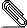# Re: [NTG-context] extra white after equation

I guess I have found the culprit in the bad placement of formulas without an
equationnumber.

The minimal example I used is that found in strc-mat.mkiv, added a [-] in the
middle one.
\setuppapersize[A6][A6]
\setupformulae[spacebefore=0pt,spaceafter=0pt]
% <HERE REDEFINED \dododoformulanumber>
\starttext
\placenamedformula[one]{first}  \startformula a = 1 \stopformula \endgraf
\placeformula[-]                   \startformula a = 2 \stopformula \endgraf
\placenamedformula     {second} \startformula a = 3 \stopformula \endgraf
\stoptext

It appears that in the case of an absent equationnumber extra stretching takes
place at the position of the (now absent) equationnumber. Placing phantom ()'s
remedies this. The effect is best seen by enabling the
\rlap{\tracedformulamode}%-line.

May I ask Hans to take a look and change it in the next beta if this indeed a
correct solution? For my suggestion for the change see below.

Hans van der Meer

On 13 nov. 2011, at 14:57, Hans van der Meer wrote:

> I find that the placement of an equation number makes a difference on the
> amount of white after the equation. Should they both give the same amount of
> whitespace? Or is the difference intentional? But then why?
> I add a minimal example.
>
>

Output (1) as in Context ver: 2011.11.04 14:15 MKIV
Output (2) with redefined \ dododoformulanumber

% lines 240+ in strc-mat.mkiv
\def\dododoformulanumber
{\ifconditional\handleformulanumber
\hbox\bgroup
% main counter
\ifconditional\insidesubformulas
% nothing
\else
\ifcase\formulasnumbermode
\ifcase\placeformulanumbermode
\dohandleformulanumbering
\or
\dohandleformulanumbering
\or
\phantom{()}% THIS GIVES CORRECT SPACING
\or
\dohandleformulanumbering
\fi
\or
\dohandleformulanumbering
\or
% nothing
\or
\dohandleformulanumbering
\fi
\fi
% subcounter
\ifconditional\insidesubformulas
\ifcase\subformulasnumbermode
% nothing
\or
\dohandlesubformulanumbering
\or
% nothing NOT CHECKED BUT PROBABLY SAME AS ABOVE
\phantom{()}% PROBABLY HERE TOO
\or
\dohandlesubformulanumbering
\fi
\fi
%\rlap{\tracedformulamode}%
\egroup
\fi}equationwhite-bad.pdfequationwhite-good.pdf
Description: equationwhite-good.pdf

___________________________________________________________________________________
___________________________________________________________________________________